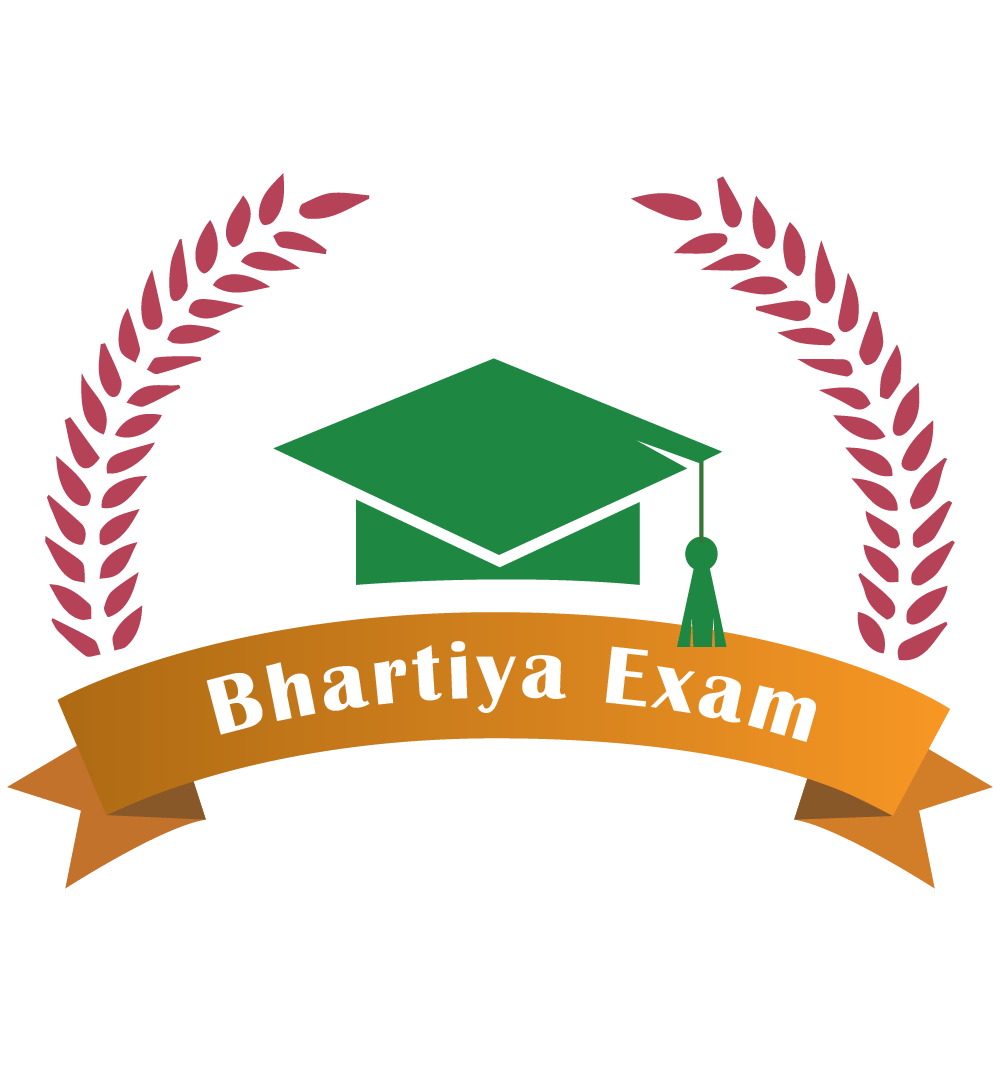आईबीपीएस, पीओ, एसएससी, और अन्य परीक्षा के लिए कंप्यूटर विज्ञान मूल बातें | Computer Science basics for IBPS,PO,SSC,and other exams

DATA REPRESENTATION -
Memory Units:

4 bit = 1 nibble
8 bit = 1 byte
1024 B = 1 KB (Kilo Byte)
1024 KB = 1 MB (Mega Byte)
1024 MB = 1 GB (Giga Byte)
1024 GB = 1 TB (Tera Byte)
1024 TB = 1 PB (Peta Byte)
1024 PB = 1 XB (Exa Byte)
1024 XB = 1 ZB (Zeta Byte)
1024 ZB = 1 YB (Yota Byte)

bit < Byte < KB < MB < GB < TB < PB < XB < ZB < YB

• bit (b)
• Byte (B)
Mbps ? mega bits per sec.
MBps ? mega Bytes per sec.

• The information you put into the computer is called Data
• Information of a computer is stored as Digital Data
• A number system defines a set of values that is used to represent Quantity
• In which number system, the modern computers are operated?

Binary Number System
• Name the most significant bit, which represent 1 and 0 for a positive number and negative number, respectively.

Sign Bit
• Which coding scheme represents data in a binary form in the computer system? ASCII, EBCDIC and Unicode are the most commonly used codes under this scheme.

Binary Coding Scheme
• EBCDIC is a 8-Bit code with 256 different representations of characters. It is mainly used in mainframe computers.
• EBCDIC stands for Extended Binary Coded Decimal Interchange Code
• In the Hexadecimal Number System each number represents a power of 16. To represent the decimal numbers, this system uses numbers from 0 to 9 and characters from A to F to represent numbers 10-15, respectively. It is commonly used as a shortcut notation for groups of four binary digits

• BCD is a method that represents the decimal digits with the help of binary digits. It takes advantage that one decimal numeral can be represented by 4-bit pattern. BCD stands for Binary Coded Decimal

• This coding system is used to represent the interval storage area of the computers. In this system, every character is represented by a combination of bits. Binary Coding System

• The Base or Radix of the decimal number system is 10
• The arithmetic operations (addition, subtraction, multiplication and division) performed on the binary numbers is called Binary Arithmetic

• What is the standard code the computer industry created to represent characters? American Standard Code for Information Interchange (ASCII)
• ASCII is a code used for standardizing the storage and transfer of information amongst various computing devices.

• It is required for representing more than 64 characters. At present, the mostly used coding systems are ASCII and EBCDIC

• Which code is also known as Reflected Code? Gray Code
• The 7-bit ASCII code is widely used for Two (0 or 1)
• In the binary language, each letter of the alphabet, each number and each special character is made up of a unique combination of Eight Bits.
GENERATIONS OF COMPUTER
• Which was the first general purpose computer, designed to handle both numeric and textual information? Universal Automatic Computer (UNIVAC) (1951)

First Generation (1940-1956) Vacuum Tubes:
• The first computers used vacuum tubes for circuitry and magnetic drums for memory, and were often enormous, taking up entire rooms.
• The UNIVAC and ENIAC computers are examples of first-generation computing devices.
• In first generation of computer, this operating system allowed only one program to run at a time and a number of input jobs are grouped for processing. It is known as Batch Processing.
Second Generation (1956-1963) Transistors:
• Transistors replaced vacuum tubes and ushered in the second generation of computers.
Third Generation (1964-1971) Integrated Circuits:

• The development of the integrated circuit was the hallmark of the third generation of computers. Transistors were miniaturized and placed on silicon chips, called semiconductors, which drastically increased the speed and efficiency of computers.

Fourth Generation (1971-Present) Microprocessors:

• The microprocessor brought the fourth generation of computers, as thousands of integrated circuits were built onto a single silicon chip.
• What in the first generation filled an entire room could now fit in the palm of the hand
• Fourth generation computers also saw the development of GUIs, the mouse and handheld devices

Fifth Generation (Present and Beyond) Artificial Intelligence:

• Fifth generation computing devices, based on artificial intelligence, are still in development, though there are some applications, such as voice recognition, that are being used today.
• In 1981 IBM introduced its first computer for the home user, and in 1984 Apple introduced the Macintosh.

Hello Friends, How do you like Bhartiya Exam? kindly tell us through your comment. Send your suggestion how to imporve BhartiyExam more. Share Bhartiya Exam with your friends, WhatsApp Group, Facebook, Gmail, Google Plus. Thank you.
दोस्तों,आप सभी को BhartiyaExam कैसी लगी,आप आपने कमेंट के माध्यम से हमें बताये, BhartiyaExam को अपने दोस्तों के साथ,व्हाट्सप ग्रुप,फेसबुक पर अधिक से अधिक शेयर करे। धन्यवाद।

Note: write a valuable comment!

{{commentObj.comment}}

{{commentObj.commentDate}}एसएससी क्लर्क परीक्षा जीके General Knowledge

## Popular General Knowledge

आईबीपीएस पीओ एसएससी और अन्य परीक्षा के लिए कंप्यूटर विज्ञान मूल बातें | Computer Science basics for IBPS PO SSC and other exams

Important General Knowledge In Hindi

सामान्य ज्ञान महत्वपूर्ण प्रश्न - Important Question